# 介绍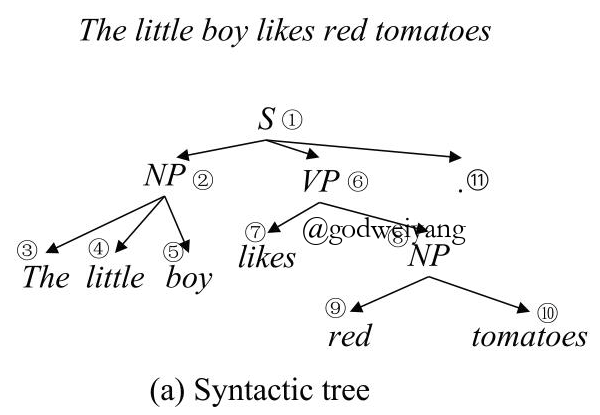Socher在2013年又提出了组合向量文法（CVG），将递归神经网络应用到了成分句法分析中，给每个短语结构赋予了向量表示。但是这种方法还是需要用到规则，采用CKY算法解码，时间效率比较低。还有一种基于CRF的神经网络句法分析方法，将离散的特征转化为了连续的特征表示。

# 基于转移系统的模型

## 自底向上的转移系统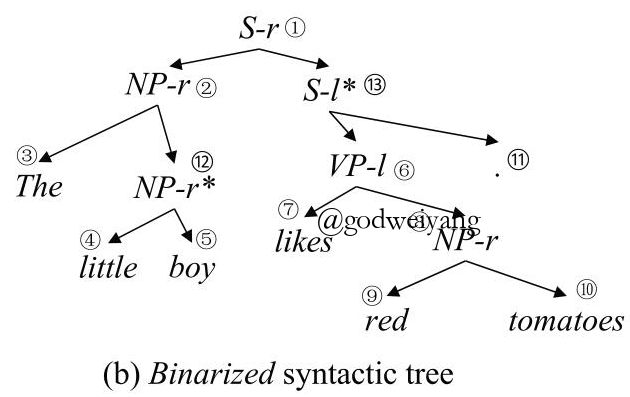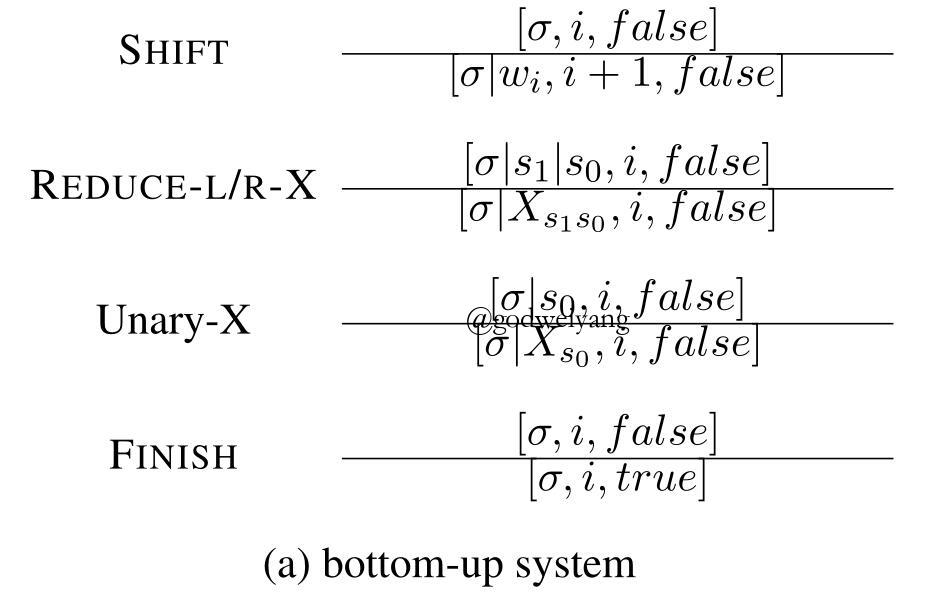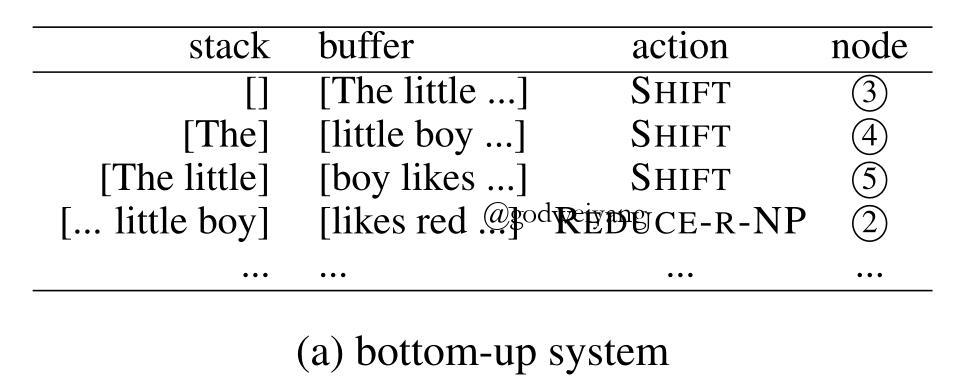## 自顶向下的转移系统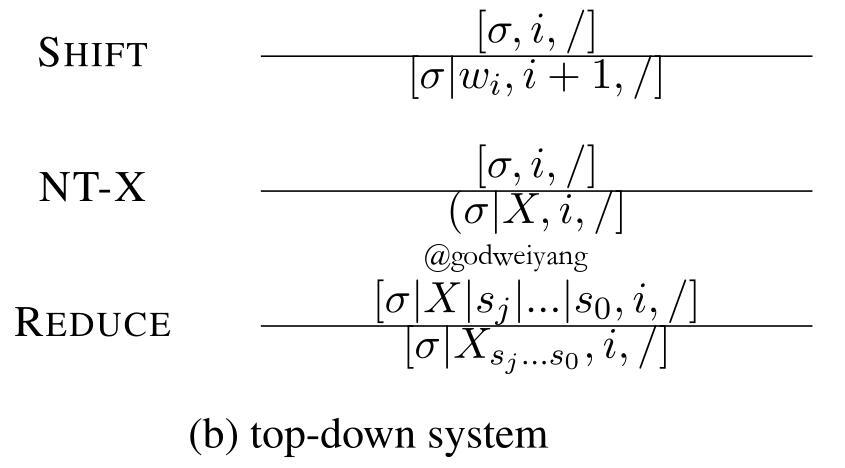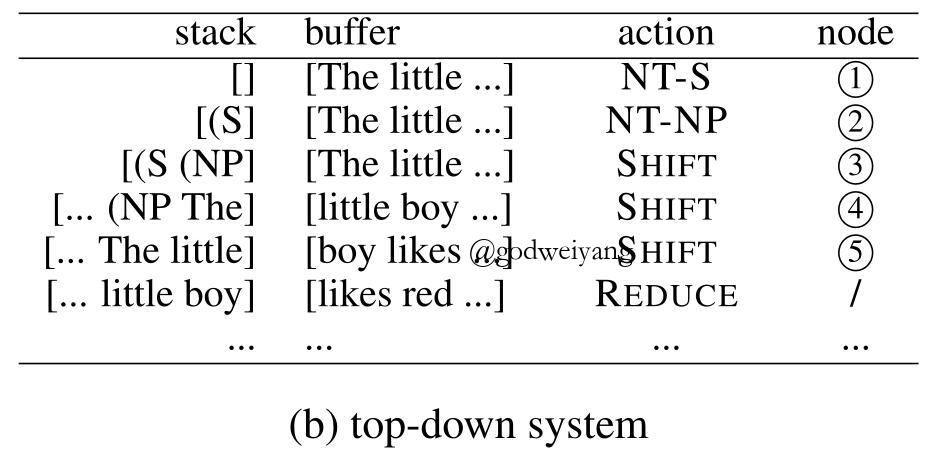## In-order转移系统

Zhang和Liu两人在2017年提出了in-order转移系统，它利用的是句法树的中序遍历（in-order）序列，首先将一个子结点SHIFT入栈，然后将父结点入栈，再不断操作直到该父结点的剩余子结点全部入栈，然后对它们进行归约。

in-order转移系统的action形式化定义如下：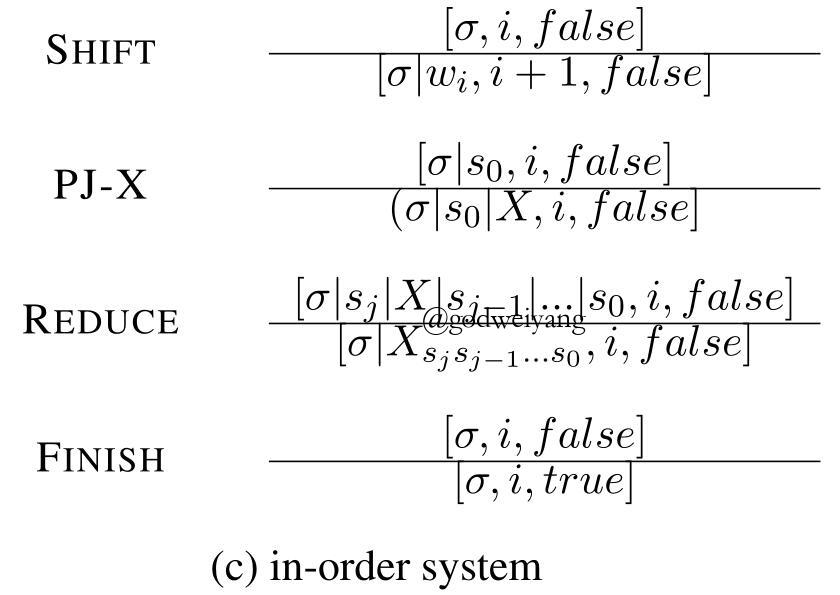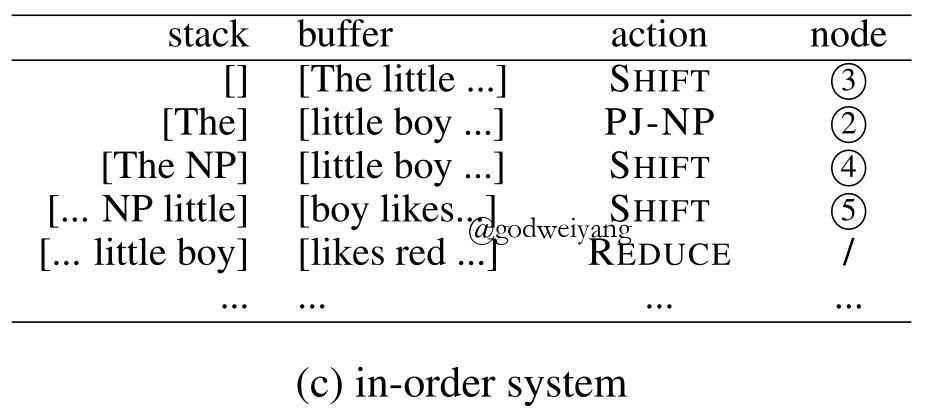in-order转移系统提出的动机也很符合人类的直觉，在你读一个句子的时候，如果你第一个看到的单词是“The”，那么你脑海中可能会想到后面紧跟着的可能是个名词短语NP，然后你继续往后看，果然验证了你的猜想，后面的单词序列是“red tomatoes”。

in-order转移系统的优点恰好结合了前面两种转移系统，既可以考虑到局部信息，又可以考虑到全局信息。

## 模型框架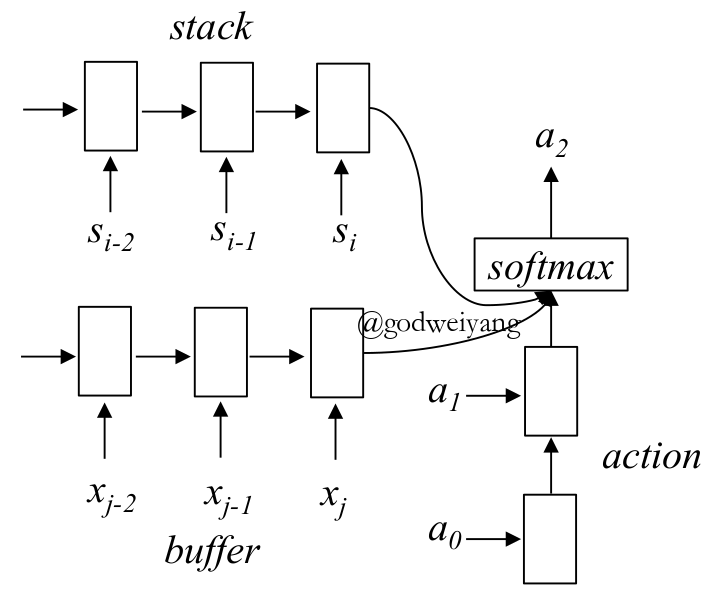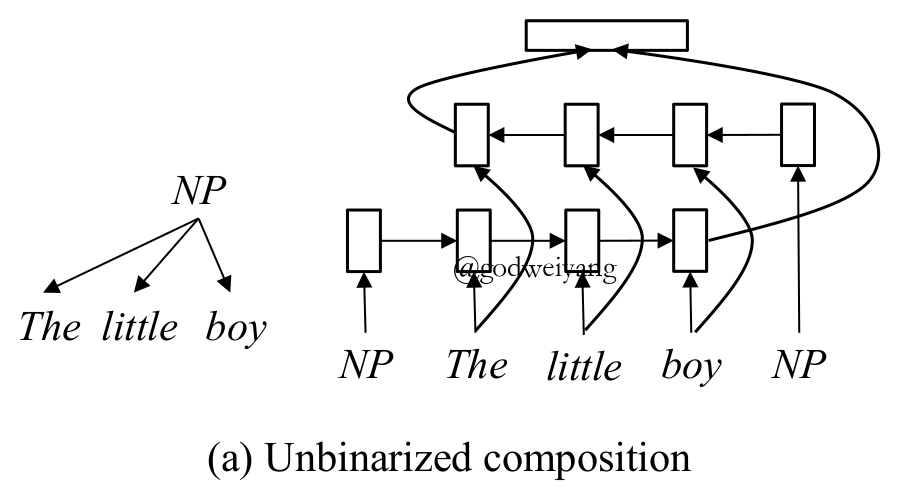## 系统改进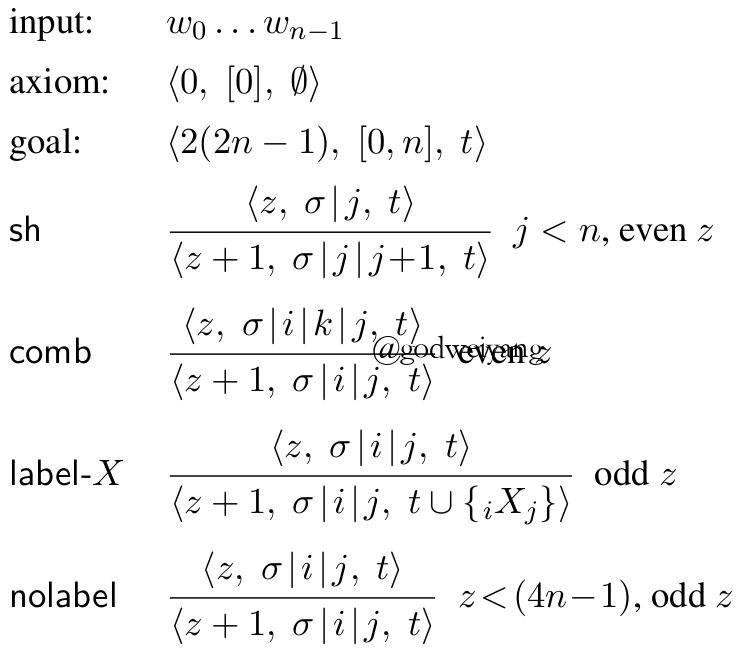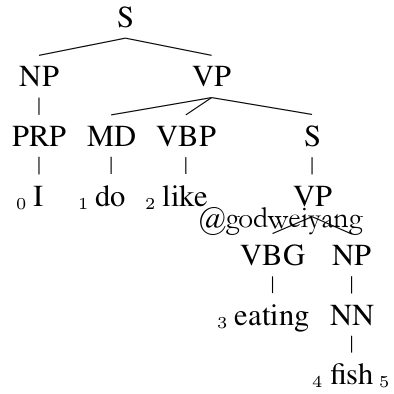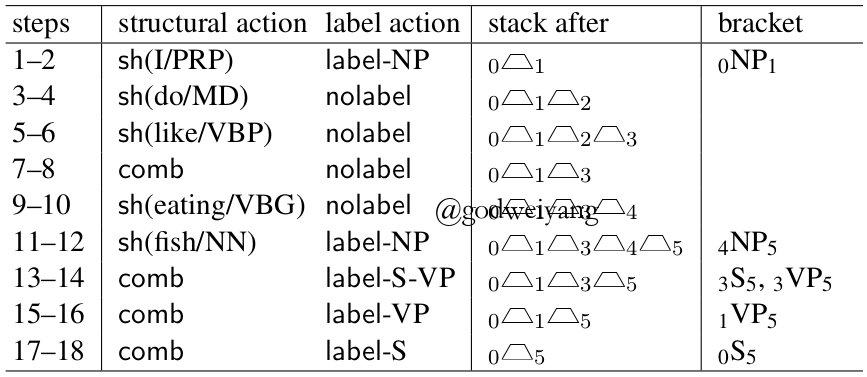RNNG是2016年提出的一种文法，全称叫做“Recurrent Neural Network Grammar”，是一种生成式模型。RNNG本质上就是自顶向下的转移系统，动作定义和之前介绍的基本一致。只是之前介绍的自顶向下的转移系统是判别式模型，每次SHIFT的单词都是buffer中给定的。而RNNG每次SHIFT的单词需要通过动作GEN(x)预测得出，最终模型对预测出来的句子分析出句法树。

$p(x) = \sum\nolimits_{y \in \mathcal{Y}(x)} {p(x,y)} = \sum\nolimits_{y \in \mathcal{Y}(x)} {q(y|x)w(x,y)} = {E_{q(y|x)}}w(x,y)$

${y^{(i)}} \sim q(y|x),i = 1,2, \ldots ,N$

$p(x) = {E_{q(y|x)}}w(x,y)\mathop \approx \limits^{MC} \frac{1}{N}\sum\limits_{i = 1}^N {w(x,{y^{(i)}})}$

Dynamic Oracle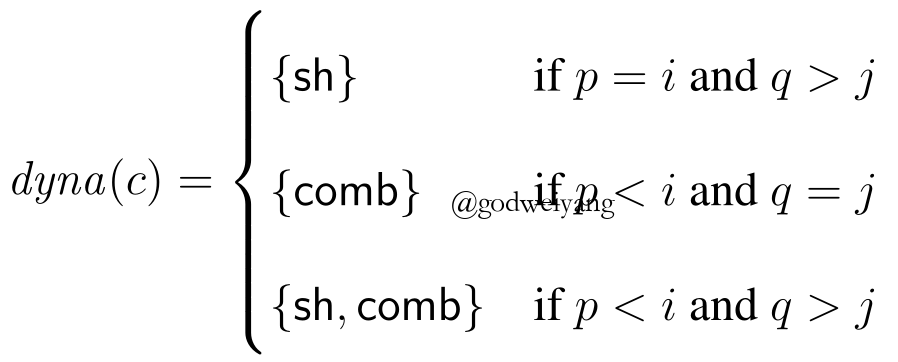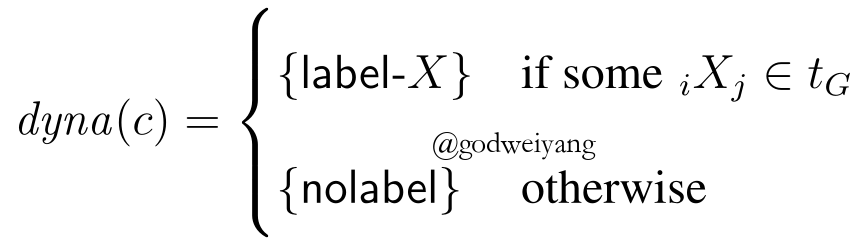$\mathcal{R}(\theta ) = \sum\limits_{i = 1}^N {\sum\limits_y {p(y|{x^{(i)}};\theta )\Delta (y,{y^{(i)}})} }$

$\begin{array}{l}\nabla \mathcal{R}(\theta ) = \sum\limits_{i = 1}^N {\sum\limits_y {p(y|{x^{(i)}})\Delta (y,{y^{(i)}})\nabla \log p(y|{x^{(i)}};\theta )} } \\ \approx \sum\limits_{i = 1}^N {\sum\limits_{y \in \mathcal{Y}({x^{(i)}})} {\Delta (y,{y^{(i)}})\nabla \log p(y|{x^{(i)}};\theta )} } \end{array}$

$\nabla p(y|{x^{(i)}};\theta ) = p(y|{x^{(i)}})\frac{ {\nabla p(y|{x^{(i)}};\theta )}}{ {p(y|{x^{(i)}};\theta )}} = p(y|{x^{(i)}})\nabla \log p(y|{x^{(i)}};\theta )$

# 编码解码模型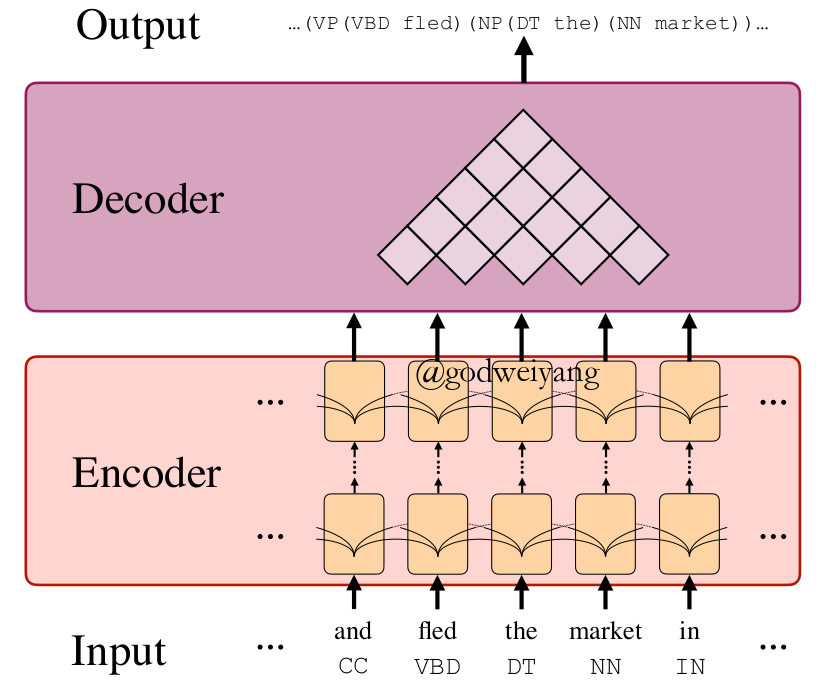## 编码器（Encoder）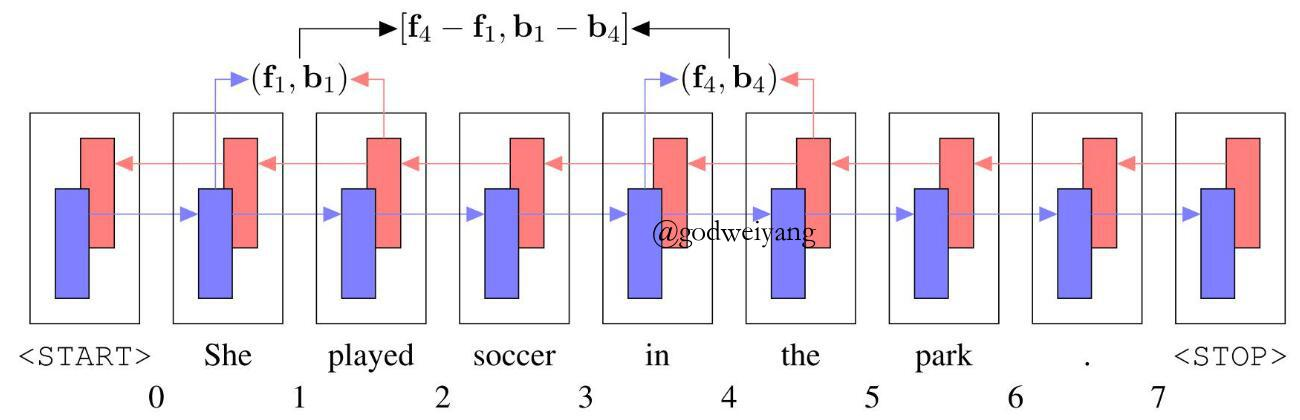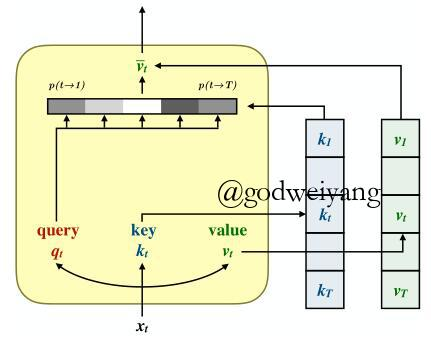$S(X) = \left[ { {\rm{softmax}}\left( {\frac{ {Q{K^{\rm{T}}}}}{ {\sqrt { {d_k}} }}} \right)V} \right]{W_O}$

$M(X) = \sum\limits_{i = 1}^8 {S(X)}$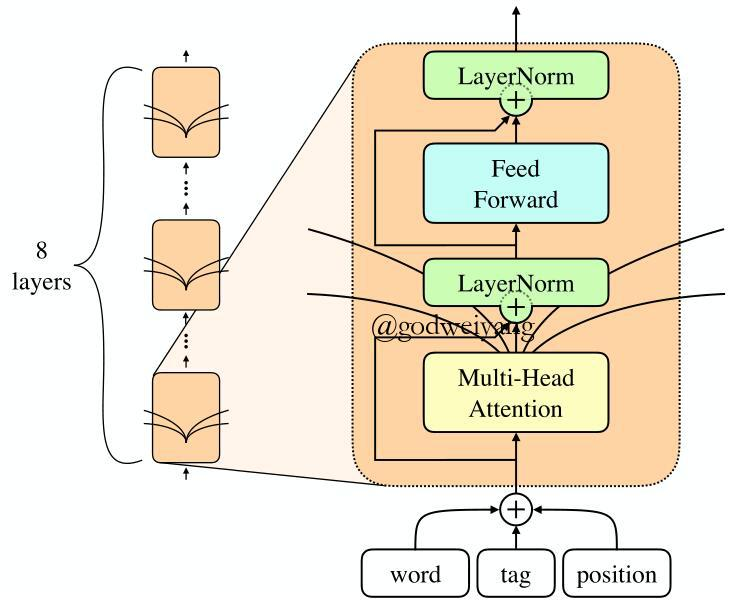## 解码器（Decoder）

${s_{tree}}(T) = \sum\limits_{(l,(i,j)) \in T} { {s_{label}}(i,j,l)}$

$s_{label}(i, j, l) = [Vg(Ws_{ij}+b)]_l$

$s_{split}(i, j, k) = s_{label}(i, j, l_{ij}) + s_{label}(i, k, l_{ik}) + s_{label}(k, j, l_{kj})$

$\mathcal{L}(\theta ) = \max \left( {0,\Delta (\hat T,T) - {s_{tree}}(T) + {s_{tree}}(\hat T)} \right)$

$\begin{array}{l}\hat l = \mathop {\arg \max }\limits_l [{s_{label}}(i,j,l)]\\\hat k = \mathop {\arg \max }\limits_k [{s_{split}}(i,k,l)]\end{array}$

# Sequence to Sequence模型

## 句法距离（Syntactic Distance）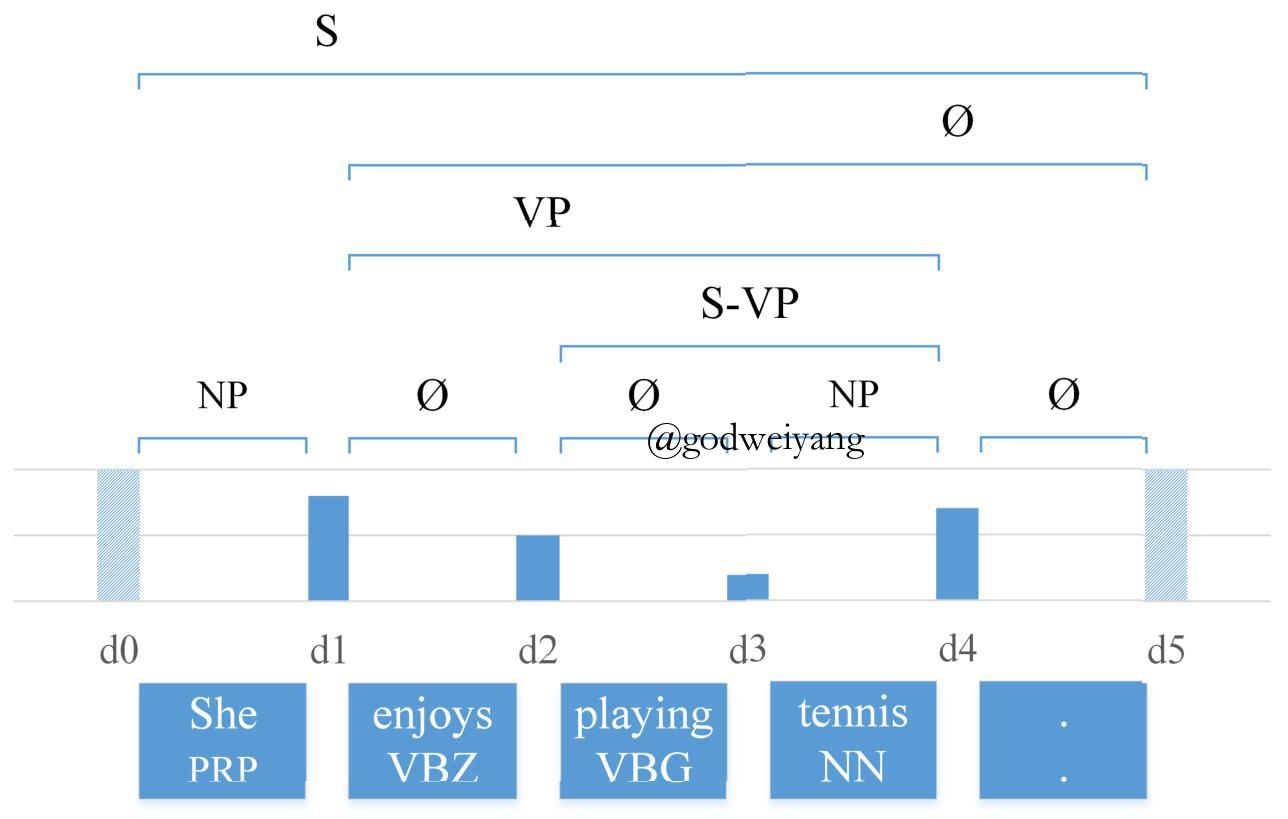# 总结

## 实验结果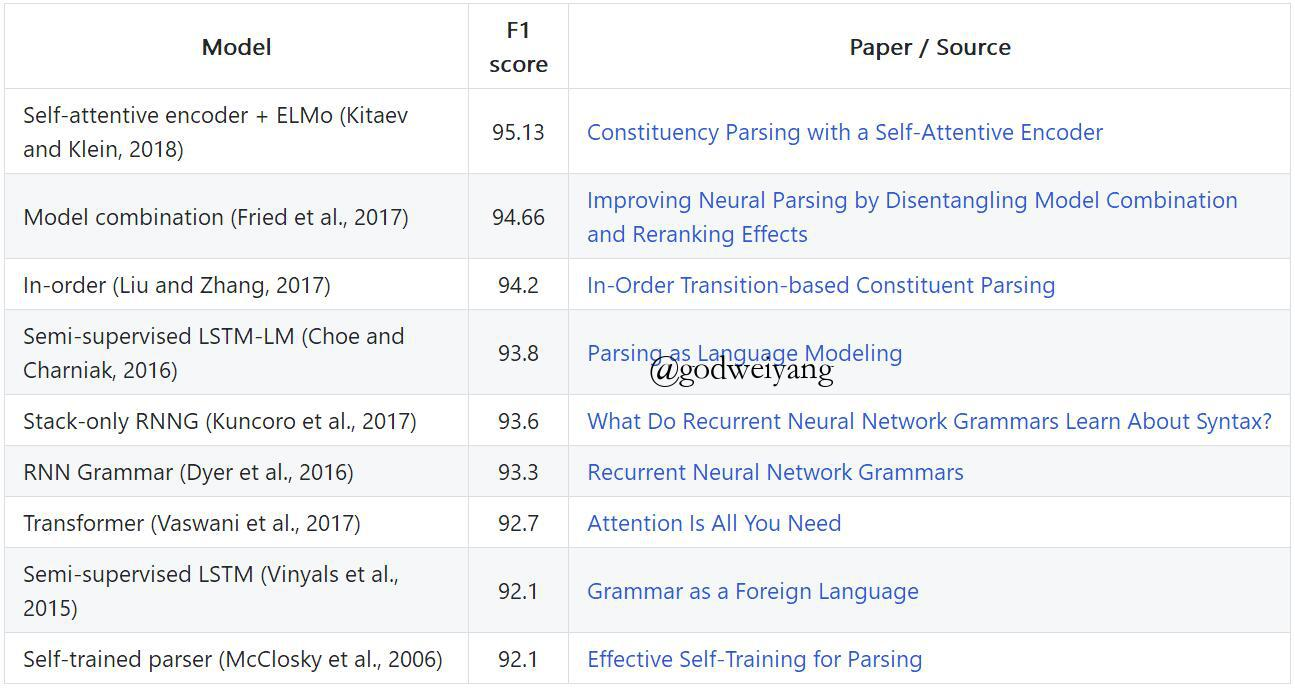# 参考文献

[ACL15] Transition-based Neural Constituent Parsing
[NAACL16] Recurrent Neural Network Grammars
[EMNLP16] Span-Based Constituency Parsing with a Structure-Label System and Provably Optimal Dynamic Oracles
[TACL17] In-Order Transition-based Constituent Parsing
[EMNLP17] Effective Inference for Generative Neural Parsing
[ACL18] Policy Gradient as a Proxy for Dynamic Oracles in Constituency Parsing
[ACL17] A Minimal Span-Based Neural Constituency Parser
[ACL18] Constituency Parsing with a Self-Attentive Encoder
[EMNLP16] Parsing as Language Modeling
[ACL18] Straight to the Tree: Constituency Parsing with Neural Syntactic Distance

上一篇给python新手的一些建议

2018-10-11Recurrent Neural Network Grammars

2018-09-02
目录